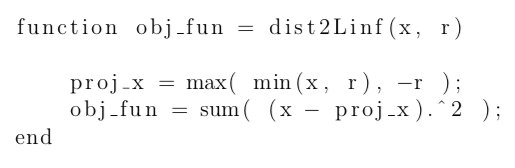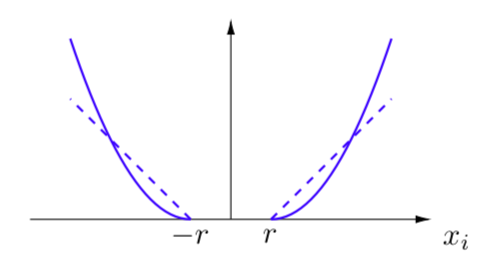# Distance from the L-infinity ball

Hi,

I need to solve an unconstrained convex minimization problem in which a part of my objective function is the squared Eucledian distance of the choice vector from the ball of the L-infinity norm with radius r>0.

The function is convex.
So far, I have been able to code it without complying to the DCP ruleset and I’ve been using optimization solvers such as fminunc on the objective function or fsolve on the gradient, which is simple to compute. However, as I do not fully trust the solutions provided by these solvers (the whole optimization problem is actually complex, but convex!), I would like to code it, if possible, according to the DCP ruleset. I tried and failed!

My codes to compute this function without complying to the DCP ruleset is:Thank you!

`0.5*proj(0) + 0.5*proj(2*r) = 0.5*r < proj(0.5*0 + 0.5*(2*r)) = proj(r) = r`
so `proj(x)` is not convex.

Am I missing something?